# Resolving forcesPage 1

#### WATCH ALL SLIDES

Slide 1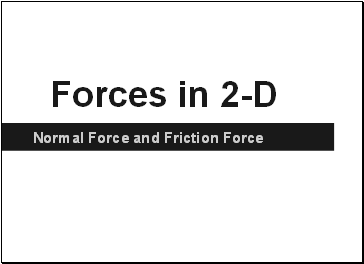Forces in 2-D

Normal Force and Friction Force

Slide 2## Normal Force

Normal Force is always perpendicular to the surface that an object is on.

Slide 3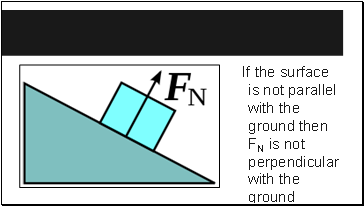If the surface is not parallel with the ground then FN is not perpendicular with the ground

Slide 4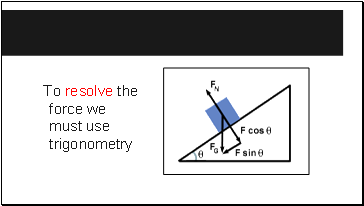To resolve the force we must use trigonometry

Slide 5Once resolved we only use the forces that are perpendicular to each

other in solving problems

Slide 6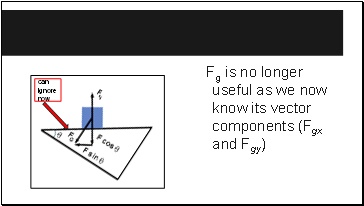Fg is no longer useful as we now know its vector components (Fgx and Fgy)

can ignore now

Slide 7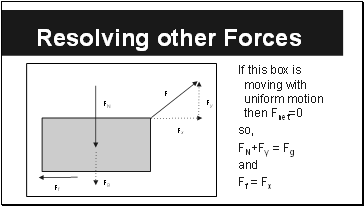## Resolving other Forces

If this box is moving with uniform motion then Fnet=0

so,

FN+Fy = Fg

and

Ff = Fx

Fg

F

FN

Fx

Fy

Ff

Slide 8Example

A 30.0kg crate experiences an applied force of 100N acting at an angle of 30O above the horizontal. What is the normal force FN on the block?

What is the horizontal acceleration?

Slide 9## Friction

A force that oppose two objects sliding against one another.

Two types

a) kinetic friction

b) static friction

Slide 10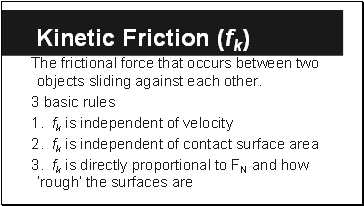## Kinetic Friction (fk)

The frictional force that occurs between two objects sliding against each other.

3 basic rules

1. fk is independent of velocity

2. fk is independent of contact surface area

3. fk is directly proportional to FN and how ‘rough’ the surfaces are

Slide 11## To find kinetic friction (fk)

fk = k FN

coefficient of friction

Slide 12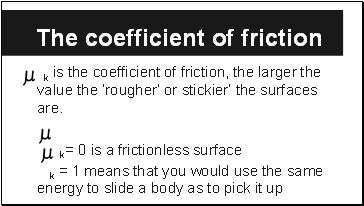## The coefficient of friction

k is the coefficient of friction, the larger the value the ‘rougher’ or stickier’ the surfaces are.

k= 0 is a frictionless surface

k = 1 means that you would use the same energy to slide a body as to pick it up

Slide 13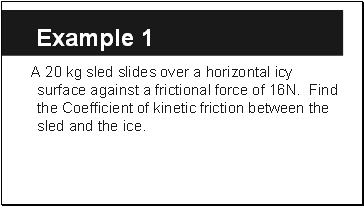Example 1

A 20 kg sled slides over a horizontal icy surface against a frictional force of 16N. Find the Coefficient of kinetic friction between the sled and the ice.

Go to page:
1  2